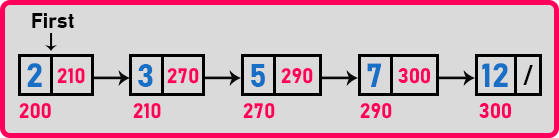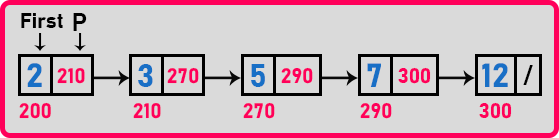# Inserting in a Sorted Linked List

## Inserting in a Sorted Linked List:

In this article, I am going to discuss Inserting in a Sorted Linked List using C Language with Examples. Please read our previous article, where we discussed Creating a Linked list using Insert using C Language with Examples.

##### Inserting in a Sorted Linked List:

Here, we will see how to insert a new node in a sorted linked list at a sorted position.This is a sorted linked list. All these keys or elements are sorted. Suppose we want to insert a new element that is ‘11’ then where should come this? ‘11’ should come after ‘7’ or before ‘12’. If we want to insert ‘4’ then where should we insert it? Between ‘3’ and ‘5’. Let us see how to do this. What should be the procedure? So let us take an example of ‘6’ and try to insert it into this sorted list.

##### Steps for Insertion in a Sorted Linked List:

The first thing we will try to find out the position where this ‘6’ should be inserted. For that, we will take the help of a pointer. Let’s take a pointer ‘p’ on the first node and start finding the position for this particular element.We will check for if (p->data < key), if yes then move ‘p’ to the next node. Here 2 is less than 6, so move ‘p’ to the next node.Here we will check again for if (p->data < key). Now ‘3’ is less than ‘6’ so move pointer ‘p’ to the next node.‘5’ is also smaller than ‘6’ again move ‘p’ to the next node.Now here ‘7’ is not less than ‘6’ so we have to insert ‘6’ before ‘7’. So, for that, we want a pointer on the previous node also i.e. ‘5’. So, we have to create a tailing pointer let’s say ‘q’ and we have to move ‘p’ and ‘q’ at the same time.

Initially, ‘q’ will point to null and ‘p’ will point to the first node. So ‘q’ will point to one node previous to the ‘p’ node. We will move both the pointer until p’s data will greater than the key value.It can be done with a single pointer also but we are doing it with 2 pointers that are ‘q’ and ‘p’. Following are the step to insert a new node:

1. Create a new node i.e. ‘t’ and store the data.
2. ‘t’ next should point on ‘q’ next.
3. ‘q’ next should point on ‘t’.Now let us write a full program to insert a node at a sorted position.

##### Program for inserting a node at a sorted position:
```#include <stdio.h>
#include <stdlib.h>

struct Node
{
int data;
struct Node *next;
}   *first = NULL, *second = NULL;

void Display(struct Node *p)
{
while (p != NULL)
{
printf ("%d ", p->data);
p = p->next;
}
}

void Create(int A[], int n)
{
int i;
struct Node *t, *last;
first = (struct Node *) malloc(sizeof (struct Node));
first->data = A;
first->next = NULL;
last = first;

for (i = 1; i < n; i++)
{
t = (struct Node *) malloc(sizeof (struct Node));
t->data = A[i];
t->next = NULL;
last->next = t;
last = t;
}
}

void SortedInsert(struct Node *p, int x)
{
struct Node *t, *q = NULL;
t = (struct Node *) malloc (sizeof (struct Node));
t->data = x;
t->next = NULL;

if (first == NULL)
first = t;
else
{
while(p && p->data < x)
{
q = p;
p = p->next;
}
if(p == first)
{
t->next = first;
first = t;
}
else
{
t->next = q->next;
q->next = t;
}
}
}

int main()
{
int A[] = { 2, 3, 5, 7, 12 };
Create (A, 5);
SortedInsert (first, 6);
Display (first);
return 0;
}
```
###### Output:In the next article, I am going to discuss Deleting from Linked List in C Language with Examples. Here, in this article, I try to explain Inserting in a Sorted Linked List using C Language with Examples and I hope you enjoy this Inserting in a Sorted Linked List using C Language with Examples article.